##### Actions

ARK method

An-stage adaptive Runge–Kutta method for the computation of approximations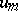for the solution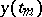of an initial-value problem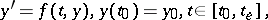is given by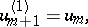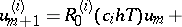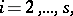Here,is an arbitrary matrix, for stability reasons usually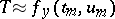. Forthe method reduces to an explicit Runge–Kutta method. The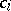are real parameters and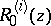,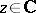, are rational approximations to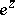for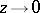. The rational matrix functions,are defined by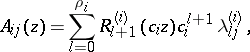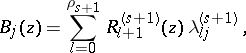with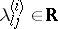and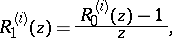The computation of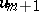requires the solution of linear systems of algebraic equations only. The coefficientsare determined to give a high order of consistency or B-consistency ([a2]). Applied to the test equation of A-stability,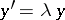with, an adaptive Runge–Kutta method with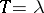yields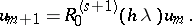By the corresponding choice of stability functions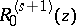, adaptive Runge–Kutta methods are A- or L-stable and therefore well suited for stiff systems (cf. Stiff differential system). Furthermore, they can be easily adapted to the numerical solution of partitioned systems, where only a subsystem of dimension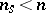is stiff. Here, by a corresponding choice ofthe dimension of the linear systems to be solved can be reduced to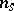[a1].